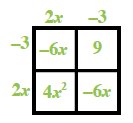### Home > AC > Chapter 13 > Lesson 13.RE1-S > Problem2-122

2-122.

For each of the following expressions, find at least three equivalent expressions. Which do you consider to be the simplest?

1. $\left(2x − 3\right)^{2} + 5$

Rewrite showing $\left(2x − 3\right)^{2}$as a product.

$\left(2x − 3\right)\left(2x − 3\right) + 5$

Multiply using a generic rectangle.Simplify the expression.

$\left(4x^{2} + \left(−6x\right) + \left(−6x\right) + 9\right) + 5\\\left(4x^{2} + \left(−12x\right) + 9\right) + 5$

Simplest Expression: $4x^{2} − 12x + 14$

1. $( \frac { 3 x ^ { 2 } y } { x ^ { 3 } } ) ^ { 4 }$

Start by writing the expression in the long form.

$\left(\frac{3\textit{x}^{2}\textit{y}}{\textit{x}^{3}}\right)\left(\frac{3\textit{x}^{2}\textit{y}}{\textit{x}^{3}}\right)\left(\frac{3\textit{x}^{2}\textit{y}}{\textit{x}^{3}}\right)\left(\frac{3\textit{x}^{2}\textit{y}}{\textit{x}^{3}}\right)$

Regroup using the Associative and Commutative properties of multiplication.

$\frac{3 \cdot 3 \cdot 3 \cdot 3 \cdot x^2 \cdot x^2\cdot x^2\cdot x^2 \cdot y \cdot y \cdot y \cdot y }{x^3 \cdot x^3 \cdot x^3 \cdot x^3 }$

$\frac{81\textit{y}^{4}}{\textit{x}^4}$